# CBSE Class 10 Maths Chapter 7 Coordinate Geometry Objective Questions

CBSE Class 10 Maths Chapter 7 Coordinate Geometry Objective Questions have become an integral part of the CBSE Board exams. Also, with the current modification of the exam pattern, more objective-type questions will be asked in the Class 10 Board exams for all subjects, including Mathematics. Here, we have compiled only the Multiple Choice Questions (MCQs) framed from various important topics in CBSE Maths Chapter 7 – Coordinate Geometry as per the Maths textbooks for CBSE Class 10. These CBSE Class 10 Maths Chapter 7 Coordinate Geometry MCQs are very useful to score good marks in Maths.

One of the main topics discussed in this chapter includes finding the area between two points whose coordinate values are provided. For instance, the area of a triangle. This chapter covers some basic concepts like the area of a triangle, rhombus, and the distance between sides and intersections. This chapter also deals with the relationship between Numerical and Geometry and their application in our daily lives. Students can download the topic-wise CBSE Class 10 Maths objective questions from the link given here.

### List of Sub-topics Covered in Chapter 7

The NCERT Solutions provided at BYJU’S are created in such a way that every step in a problem is explained clearly and in detail. The CBSE Class 10 examination often asks questions, either directly or indirectly, from the NCERT textbooks. These CBSE Class 10 Maths Chapter 7 – Coordinate Geometry Objective Questions with Solutions is also very helpful for the students to prepare for the exams. Following a change in the exam pattern, more MCQs are expected to be included in the exam paper in this academic year.

7.1 Basics Revisited (5 MCQs from the Topic)

7.2 Distance Formula (5 MCQs from the Topic)

7.3 Section Formula (5 MCQs from the Topic)

7.4 Area from Coordinates (5 MCQs from the Topic)

## Download CBSE Class 10 Maths Chapter 7 – Coordinate Geometry Objective Questions Free PDF

### Basics Revisited

1. What will be the reflection of the point (4, 5) about the x-axis in the fourth quadrant?
1. (4, 5)
2. (4, -5)
3. (-4, -5)
4. (-4, 5)

Solution: X-axis will act as a plane mirror, and this point will form an image, following the sign convention, at (4, -5) in the fourth quadrant.

1. Point P lies on the line 3x + 4y – 12 = 0. If the x-coordinate of P is a, then its y-coordinate is ______.
1. (12-3a) / 4
2. (12-4a)/ 3
3. (12+3a)/4
4. (3a-12)/4

Solution: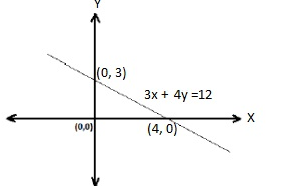The points on the line should satisfy the equation of the line.

So, the point P (a, y) satisfies the equation.

Hence,

3x+ 4y – 12 = 0

3(a) + 4(y) -12 = 0

4y = 12-3a

Y = (12-3a) / 4

1. If point P lies on the line y = -1, find the following a) Its y-coordinate, b) Its x-coordinate
1. y = -1, x can be any real number
2. y = 1, x = -1
3. x = -2, y = -1
4. x = -1, y = -1

Answer: (A) y = -1, x can be any real number

Solution: Since it is given that the point P lies on the line y = -1, its y-coordinate will be -1, and the x-coordinate can be any real number.

1. Find the values of k if the points A (k+1, 2k), B (3k, 2k+3), and C (5k-1, 5k) are collinear.
1. k = 5, 1/5
2. k = 4, 1/4
3. k = 3, 1/3
4. k = 2, 1/2

Answer: (D) k = 2, 1/2

Solution: We know that if three points are collinear, then the area of the triangle formed by these points is zero.

Since the points A (k+1,2k), B (3k, 2k+3) and C (5k-1,5k) are collinear,

The area of Δ ABC = 0.

⇒ ½ [x1(y2−y3) +x2(y3−y1) +x3(y1−y2)] =0

Multiplying the above expression by 2, we get

[x1(y2−y3) +x2(y3−y1) +x3(y1−y2)] =0

Here, x1=k+1, x2=3k, x3=5k−1

And y1=2k, y2=2k+3, y3=5k

⇒[(k+1)(2k+3−5k)+3k(5k−2k)+(5k−1)(2k−(2k+3))]=0⇒[(k+1)(3−3k)+3k(3k)+(5k−1)(2k−2k−3)]=0

⇒ [−3k2+3k−3k+3+9k2−15k+3] =0

⇒ (6k2−15k+6) =0

⇒6k2−15k+6=0

Dividing the equation by 3, we get

⇒2k2−5k+2=0

⇒2k2−4k−k+2=0 [by factorisation method]

⇒2k(k−2)−1(k−2)=0

⇒ (k−2) (2k−1) =0

If k – 2 = 0, then k = 2

If 2k – 1, then k = 1/2

∴ k=2, 1/2

Hence, the required values of k are 2 and 1/2.

1. Name the type of triangle formed by the points A (-5, 6), B (-4,-2), and C (7, 5).
1. Equilateral triangle
2. Scalene triangle
3. Isosceles triangle
4. Right-angled triangle

Solution: To find the type of triangle, first, we determine the length of all three sides and see if the condition of the triangle is satisfied by these sides.

Now, using the distance formula between two points,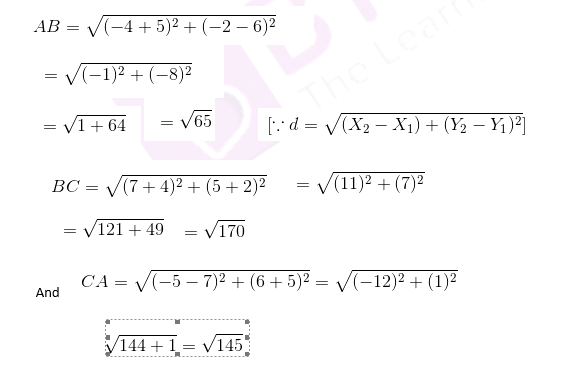We see that AB≠BC≠CA

And ΔABC does not satisfy the Pythagoras theorem

Hence, the required triangle is scalene because all sides have different lengths.

### Distance Formula

1. The distance of the point (–2, –2) from the origin is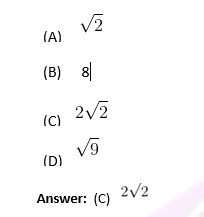Solution: Let the origin be O and the point A be (-2, -2)

Using the distance formula,

OA2 = (22 + 22)

OA2 = 8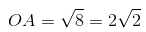1. The points on the X-axis at a distance of 10 units from (11, –8) are:
1. (5, 2) (17, 0)
2. (5, 0) (17, 0)
3. (6, 0) (17, 0)
4. (5, 0) (16, 0)

Answer: (B) (5, 0) (17, 0)

Solution: Any point on the x-axis is of the form (x, 0)

Let the point be (x,0)

Using the distance formula,

(x−11)2+82=102

x2−22x+121+64=100

x2−22x+85=0,

By factorising, we get,

x2−17x−5x+85=0

(x−17)(x−5)=0

x=17, 5

Hence, the points are (17,0) and (5,0).

1. The point on the x-axis, which is equidistant from (2, –5) and (–2, 9), is:
1. (7, 0)
2. (-7, 0)
3. (2, 0)
4. (-2, 0)

Solution: We know that a point on the x-axis is of the form (x, 0). Let the point on the x-axis be P(x, 0), and the given points are A (2, -5) and B (-2, 9).

Now,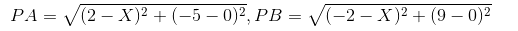Since PA = PB,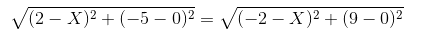⇒ (2−x)2 + (−5−0)2 = (−2−x)2 + (9−0)2

⇒ 4 – 4x + x2 + 25 = 4 + 4x + x2 + 81

⇒ – 8x = 56

⇒ x = -7

Hence, the required point is (-7, 0)

1. The points (6. 6), (0, 6), and (6, 0) are the vertices of a right triangle, as shown in the figure. Find the distance between its centroid and circumcentre.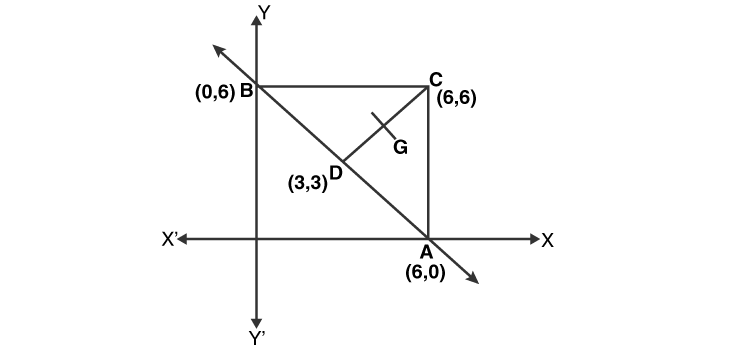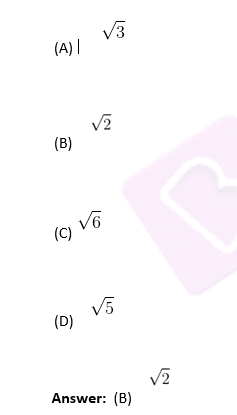Solution: Circumcentre = midpoint of AB

= [(X1 + X2)/2, (Y1 + Y2)/2]

= [(6+0)/2, (6+0)/2]

= (3, 3)

Centroid (G) = [(X1 + X2+ X3)/3, (Y1+Y2+ Y3)/3]

= [(6+0+6)/3, (0+6+6)/3]

= (4, 4)

∵ The distance between two points (x1, y1) and (x2, y2) is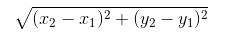∴ The distance between the centroid and circumcentre is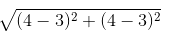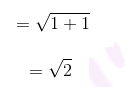1. The distance between the points (a, b) and (–a, –b) is: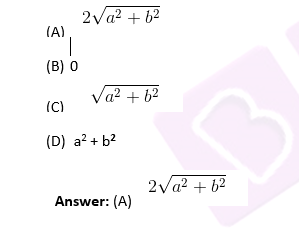Solution: Let A (a, b) and B (-a,-b) be the two points and ’d’ be the distance between them.

By using the distance formula, we get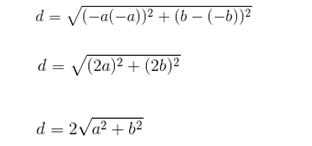### Section Formula

1. Mid-point of the line segment joining the points (–5, 4) and (9, –8) is:
1. (-2,2)
2. (7,-6)
3. (2,-2)
4. (-7,6)

Solution: Midpoint of a line segment joining (x1, y1) and (x2, y2) is [(x1+ x2)/2, (y1+y2)/2]

∴ Mid-point of the line-segment joining the points (–5, 4) and (9, –8) = [(9-5)/2, (4-8)/2] = (2, -2)

1. The line x + y = 10 divides line segment AB in the ratio a:1. Find the value of a.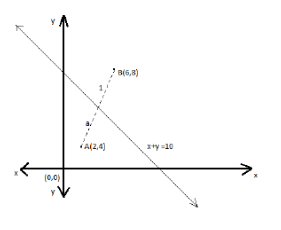1. ½
2. 1
3. 2
4. 3

Solution: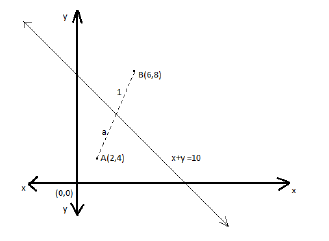The point, say P(x, y), divides the line AB into the ratio a:1.

The equation for the point that divides a line in the ratio m:n is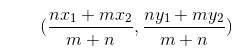where (x1, y1) and (x2, y2) are the coordinates of the endpoints of the line segment.

Applying the formula, we get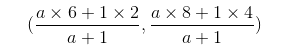This point lies on the line x + y = 10, so substitute the points in the equation for the line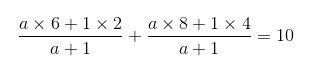6a + 2 + 8a + 4 = 10(a+1)

4a = 4

a = 1

1. Points A (1, 2) and B (3, 4) are two ends of a line segment. Find the point which divides AB in the ratio 3:4.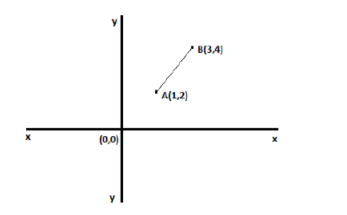1. (4,3)
2. (2,3)
3. 15/7, 22/7
4. 13/7, 20/7

Solution: The coordinates for the point that divides a line in the ratio m:n is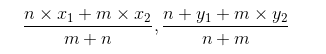Substituting in the equation, we get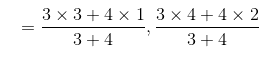= 13/7, 20/7

Therefore, the point which divides the line segment in the ratio 3:4 is 13/7, 20/7.

1. Find the point (x,y) that divides the join of A(3,6) and B(7,10) in the ratio 3:1
1. (6, 9)
2. (4, 5)
3. (8, 9)
4. None of these

Solution: If (x, y) divides the join of A(x1, y1) and (x2, y2) in the ratio m:n

Then,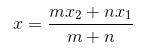and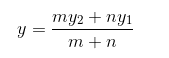Here, x1=3, x2=7, y1=6, y2=10, m = 3 and n = 1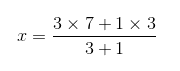and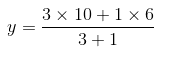x = 6 and y = 9

Therefore, the point is (6, 9).

1. If (1, 2), (4, y), (x, 6), and (3, 5) are the vertices of a parallelogram taken in order, find x and y.
1. (2, 3)
2. (4, 3)
3. (6, 3)
4. (2, 5)

Solution: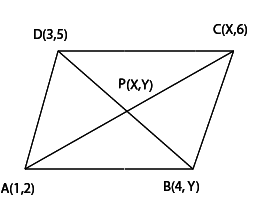The given points are: A(1,2), B(4, y), C(x, 6), and D(3,5)

Since the diagonals of a parallelogram bisect each other,

The coordinates of P are:

X= (x+1)/ 2 = (3+4)/2

⇒x+1=7⇒x=6

Y = (5+Y)/2= (6+2)/2

⇒5+y=8⇒y=3

∴ The required values of x and y are:

x=6, y=3.

### Area from Coordinates

1. The area of a quadrilateral whose vertices taken in order are (–4, –2), (–3, –5), (3, –2), and (2, 3) is _______.
1. 26 sq. units
2. 28 sq. units
3. 30 sq. units
4. 27 sq. units

Solution: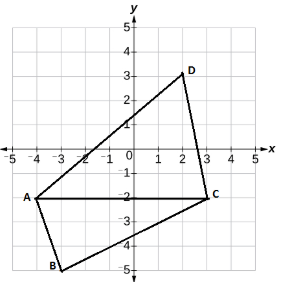Consider the points A(−4,−2),B(−3,−5),C(3,−2) and D(2,3).

Area of a triangle having coordinates (x1, y1), (x2, y2), (x3, y3) is given by

½ × |x1 (y2−y3) +x2(y3−y1) +x3(y1−y2)|

∴ The area of ΔABC

= 1/2 | (-4) (-5 + 2) -3(-2 + 2) + 3(-2 + 5)|

=  1/2|20−8−6+15|=21/2 = 10.5 sq. units

Similarly, the area of  ΔACD

= 1/2| (-4) (-2 – 3) +3(3 + 2) + 2(-2 + 2)|

= 1/2|20+15|=35/2 = 17.5 sq. units

Now, area of quadrilateral ABCD = Area (ΔABC) + Area (ΔACD) = (10.5 + 17.5) sq. units = 28 sq. units.

1. If the points (a, 0), (0, b) and (1, 1) are collinear, then which of the following is true?
1. (1/a) + (1/b)= 2
2. (1/a) + (1/b)= 1
3. (1/a) + (1/b)= 0
4. (1/a) + (1/b)= 4

Answer: (B) (1/a) + (1/b) = 1

Solution: Area of a triangle formed by (x1, y1), (x2, y2), (x3, y3) = 1/2 [x1(y2 – y3) + x2(y3 – y1)

+ x3(y1 – y2)]

For 3 points to be collinear, the area of the triangle should be zero:

⇒1/2[a (b−1) + 0(1−0) + 1(0−b)] =0

⇒1/2[a (b−1) +1(0−b)] =0

⇒ab=a+b

⇒ (1/a) + (1/b) = 1

1. If 2 triangles have the same height, the ratio of their areas is equal to
1. The ratio of any 2 sides
2. The ratio of their corresponding bases
3. The ratio of their heights
4. 1

Answer: (B) The ratio of their corresponding bases

Solution: Area of triangle 1 / Area of triangle 2 = (½ × Base 1× height) / (½ × Base 2× height) = base 1/ base 2

1. The area of triangle with vertices A(x1,y1),B(x2,y2) and C(x3,y3) is: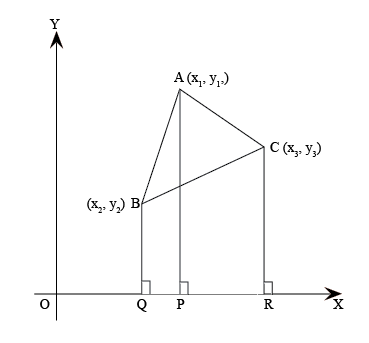(A) 1/2[x2(y2−y3) +x3(y3−y1) +x2(y1−y2)]

(B) 1/2[x1(y2−y3) +x3(y3−y1) +x1(y1−y2)]

(C) 1/2[x1(y2−y3) +x2(y3−y1) +x3(y1−y2)]

(D) All of the above

Solution: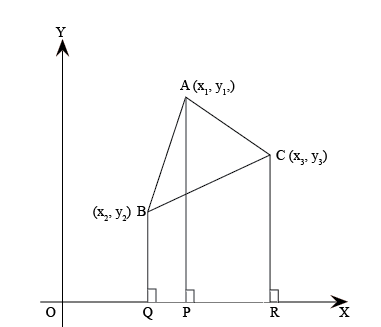Let ABC be any triangle whose vertices are A(x1, y1), B(x2, y2) and C(x3, y3). Draw AP, QR and CR perpendiculars from A, B and C, respectively, to the x-axis. Clearly, ABQP, APRC and BQRC are trapezia.

Now from the figure, it is clear that

Area of △ABC = Area of trapezium ABQP + Area of trapezium APRC – Area of trapezium BQRC.

Area of a trapezium =1/2 (Sum of parallel sides)(Distance between the parallel sides)

Therefore,

The area of ΔABC=1/2(BQ+AP)QP+1/2(AP+CR)PR−1/2(BQ+CR)QR

=1/2(y2+y1)(x1−x2)+1/2(y3+y1)(x3−x1)−1/2(y2+y3)(x3−x2)

=1/2[x1(y2−y3)+x2(y3−y1)+x3(y1−y2)]

Thus, the area of ΔABC is the numerical value of the expression 1/2[x1(y2−y3)+x2(y3−y1)+x3(y1−y2)].

1. P(x, y), A (3, 4) and B (5, -2) are the vertices of triangle PAB, such that |PA|=|PB| and area of ΔPAB=10 sq. units, and PA = k√5 units.  Find the value of k.
1. 2
2. 1
3. √5
4. 3

Solution: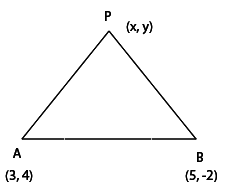Given, |PA|=|PB|

∴ PA2=PB2

⇒ (x−3)2+ (y−4)2 = (x−5)2+(y+2)2

⇒x2−6x+9+y2−8y+16=x2−10x+25+y2+4y+4

⇒4x−12y=4⇒x−3y=1…(i)

The area of ΔPAB=1/2|x (4+2) +3(−2−y) +5(y−4)|

⇒10=1/2|6x−6−3y+5y−20|

⇒20=6x+2y−26⇒6x+2y−26=20 or 6x+2y−26=−20

⇒6x+2y=46 or 6x+2y=63x+y=23… (2)

or 3x+y=3… (3)

By solving (1) and (3), we get

x=1, y=0

Solving (1) and (2), we get

x=7, y=2

∴ Coordinates of P are (7, 2) and (1, 0)

Length of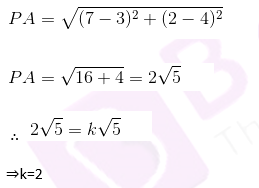Also, the Length of PA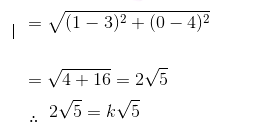⇒k = 2

Each question is provided with the answer and a detailed solution. Students are, however, advised to solve these questions on their own before referring to the answers in order to self-assess their performance. Also, find here some CBSE Class 10 Maths Chapter 7 Extra MCQs.

## CBSE Class 10 Maths Chapter 7 Extra MCQs

1. The points (-5, 1), (1, p) and (4, -2) are collinear if the value of p is _____.
(a) -1
(b) 2
(c) 1
(d) 3

2. What are the points (1,1), (-2, 7) and (3, -3)?
(a) vertices of an isosceles triangle
(b) collinear
(c) vertices of an equilateral triangle
(d) none of the above

BYJU’S, India’s top online education platform, provides NCERT study materials online. To get access to all the CBSE study materials, visit BYJU’S website or download BYJU’S – The Learning App.